Home > CC3 > Chapter 9 > Lesson 9.2.2 > Problem9-78

9-78.
1.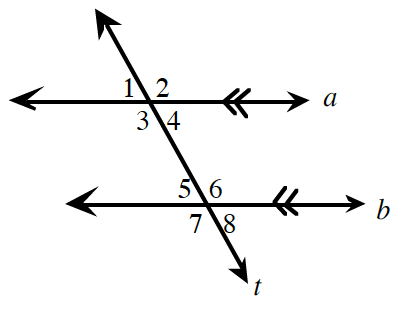Solve for x. Each part is a separate problem. Homework Help ✎

1. If m∠1 = 3x – 18° and m∠5 = 2x + 12°, find x.

2. If m∠3 = 4x – 27° and m∠6 = x + 39°, find x.

3. If m∠4 = 49° and m∠6 = 5x + 41°, find x.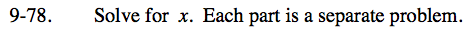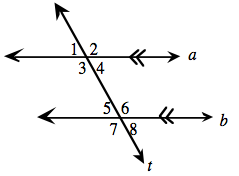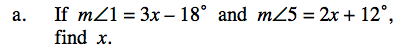Angles 1 and 5 are corresponding angles. Since lines a and b are parallel, the angles are equal.

x = 30°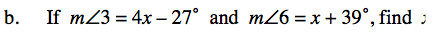Angles 3 and 6 are alternate interior angles. Since lines a and b are parallel, the angles are equal.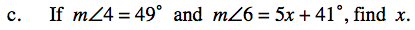Angles 4 and 6 are supplementary because the lines cut by the transversal are parallel.

49 + (5x + 41) = 180